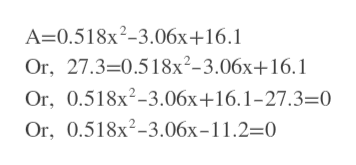The assets A of public pension funds (in billions of dollars) can be approximated by the function A=0.518x2-3.06x+16.1, where x=3 corresponds with the year 2003. What were the assets in 2013? What year(s) produced \$27.3 Billion?

Question

The assets A of public pension funds (in billions of dollars) can be approximated by the function A=0.518x2-3.06x+16.1, where x=3 corresponds with the year 2003.

What were the assets in 2013?

What year(s) produced \$27.3 Billion?

Step 1

x=3 corresponds to 2003.

So, 2013 is 3+(2013-2003)= 3+10=13 year

Step 2

We will plug x=13 in the given equation and then we find A.

Answer: 63.862 billions of dollars.

Step 3

For A=27.3 we have to find x now.

We ...help_outlineImage TranscriptioncloseA-0.518x2-3.06x+16.1 Or, 27.3-0.518x2-3.06x+16.1 Or, 0.518x2-3.06x+16.1-27.3=0 Or, 0.518x2-3.06x-11.2=0 fullscreen

Want to see the full answer?

See Solution

Want to see this answer and more?

Our solutions are written by experts, many with advanced degrees, and available 24/7

See Solution
Tagged in

Other